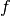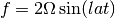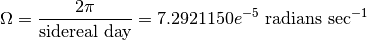# extras¶

seawater.extras.dist(lat, lon, units='km')

Calculate distance between two positions on globe using the “Plane Sailing” method. Also uses simple geometry to calculate the bearing of the path between position pairs.

Parameters: lat : array_like decimal degrees (+ve N, -ve S) [- 90.. +90] lon : array_like decimal degrees (+ve E, -ve W) [-180..+180] units : string, optional default kilometers dist : array_like distance between positions in units phaseangle : array_like angle of line between stations with x axis (East). Range of values are -180..+180. (E=0, N=90, S=-90)

References

 [R23] The PLANE SAILING method as described in “CELESTIAL NAVIGATION” 1989 by Dr. P. Gormley. The Australian Antarctic Division.

Examples

>>> import seawater as sw
>>> sw.dist(0, [-179, 180])
(array([ 111.12]), array([ 180.]))
>>> lon = [35, 35]
>>> lat = [41, 40]
>>> sw.dist(lat, lon)
(array([ 111.12]), array([-90.]))
>>> # Create a distance vector.
>>> lon = np.arange(30,40,1)
>>> lat = 35
>>> np.cumsum(np.append(0, sw.dist(lat, lon, units='km')))
array([   0.        ,   91.02417516,  182.04835032,  273.07252548,
364.09670065,  455.12087581,  546.14505097,  637.16922613,
728.19340129,  819.21757645])

seawater.extras.f(lat)

Calculates the Coriolis factordefined by:where:Parameters: lat : array_like latitude in decimal degrees north [-90..+90]. f : array_like Coriolis factor [s -1]

References

 [R24] S. Pond & G.Pickard 2nd Edition 1986 Introductory Dynamical Oceanography Pergamon Press Sydney. ISBN 0-08-028728-X
 [R25] A.E. Gill 1982. p.54 Eqn. 3.7.15 “Atmosphere-Ocean Dynamics” Academic Press: New York. ISBN: 0-12-283522-0
 [R26] Groten, E., 2004: Fundamental Parameters and Current (2004) Best Estimates of the Parameters of Common Relevance to Astronomy, Geodesy, and Geodynamics. Journal of Geodesy, 77, pp. 724-797.

Examples

>>> import seawater as sw
>>> sw.f(45)
0.00010312445296824608

seawater.extras.satAr(s, t)

Solubility (saturation) of Argon (Ar) in sea water.

Parameters: s : array_like salinity [psu (PSS-78)] t : array_like temperature [℃ (ITS-90)] satAr : array_like solubility of Ar [ml l -1]

References

 [R27] Weiss, R. F. 1970. The Solubility of Nitrogen, Oxygen and Argon in Water and Seawater Deep-Sea Research Vol. 17, p. 721-735. doi:10.1016/0011-7471(70)90037-9

Examples

>>> # Data from Weiss 1970.
>>> import seawater as sw
>>> from seawater.library import T90conv
>>> t = T90conv([[ -1, -1], [ 10, 10], [ 20, 20], [ 40, 40]])
>>> s = [[ 20, 40], [ 20, 40], [ 20, 40], [ 20, 40]]
>>> sw.satAr(s, t)
array([[ 0.4455784 ,  0.38766011],
[ 0.33970659,  0.29887756],
[ 0.27660227,  0.24566428],
[ 0.19861429,  0.17937698]])

seawater.extras.satN2(s, t)

Solubility (saturation) of Nitrogen (N2) in sea water.

Parameters: s : array_like salinity [psu (PSS-78)] t : array_like temperature [℃ (ITS-90)] satN2 : array_like solubility of N2 [ml l -1]

References

 [R28] Weiss, R. F. 1970. The Solubility of Nitrogen, Oxygen and Argon in Water and Seawater Deep-Sea Research Vol. 17, p. 721-735. doi:10.1016/0011-7471(70)90037-9

Examples

>>> # Data from Weiss 1970.
>>> import seawater as sw
>>> from seawater.library import T90conv
>>> t = T90conv([[ -1, -1], [ 10, 10], [ 20, 20], [ 40, 40]])
>>> s = [[ 20, 40], [ 20, 40], [ 20, 40], [ 20, 40]]
>>> sw.satN2(s, t)
array([[ 16.27952432,  14.00784526],
[ 12.64036196,  11.01277257],
[ 10.46892822,   9.21126859],
[  7.78163876,   6.95395099]])

seawater.extras.satO2(s, t)

Solubility (saturation) of Oxygen (O2) in sea water.

Parameters: s : array_like salinity [psu (PSS-78)] t : array_like temperature [℃ (ITS-68)] satO2 : array_like solubility of O2 [ml l -1 ]

References

 [R29] Weiss, R. F. 1970. The Solubility of Nitrogen, Oxygen and Argon in Water and Seawater Deep-Sea Research Vol. 17, p. 721-735. doi:10.1016/0011-7471(70)90037-9

Examples

>>> # Data from Weiss 1970.
>>> import seawater as sw
>>> from seawater.library import T90conv
>>> t = T90conv([[ -1, -1], [ 10, 10], [ 20, 20], [ 40, 40]])
>>> s = [[ 20, 40], [ 20, 40], [ 20, 40], [ 20, 40]]
>>> sw.satO2(s, t)
array([[ 9.162056  ,  7.98404249],
[ 6.95007741,  6.12101928],
[ 5.64401453,  5.01531004],
[ 4.0495115 ,  3.65575811]])

seawater.extras.swvel(length, depth)

Calculates surface wave velocity.

length : array_like
wave length
depth : array_like
water depth [meters]
Returns: speed : array_like surface wave speed [m s -1]

Examples

>>> import seawater as sw
>>> sw.swvel(10, 100)
3.9493270848342941